#!/usr/local/bin/perl # # Math Journey CGI game # \$Id\$ # Copyright (c) 2001, Quia Corporation. All rights reserved. # # created by pmishkin in 1999 # # This CGI is invoked from the mathjourney.html page. Since it is our only # Perl CGI game and we are not planning to add any other such games, some # portions of it need not be maintained. # use BootstrapIXLPerlEnvironment; # include this first use quia::util::GeneralUtils ; use quia::conf::JavaQuiaConf ; use quia::activities::mathjourney::ixlbanner ; &ReadParse(*in) ; srand; \$num_skills = &get_num_skills; \$html_content = 'Content-type: text/html; charset=utf-8'; # fixing bug 12173, ltang, 02/01/2008 if (\$in{"missed_flag"} eq "y") { \$skillset = \$in{"skillset"}; @skill_array = split(/-/, \$skillset); \$num_missed = \$in{"num_missed"}; \$segment_id = \$in{"segment_id"}; if (\$num_missed == 3) { \$html = &get_over_html(\$segment_id); print \$html_content . "\n\n"; print \$html . "\n\n"; exit; } } elsif (\$in{"start_flag"} eq "y") { \$skillset = ""; for (\$i = 0; \$i < \$num_skills; \$i++) { if (\$in{&get_skill_code(\$i)} eq "y") { push @skill_array, \$i; \$skillset .= "-" . \$i; } } \$skillset = substr(\$skillset, 1); \$segment_id = 0; \$num_missed = 0; if (\$skillset eq "") { \$msg = 'Error - No Skills Selected

You didn\'t select any skills.

Press your browser\'s BACK button and select the skills you want to practice.
 You win!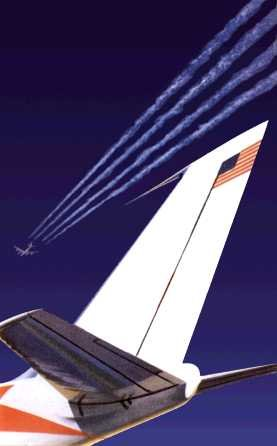Distance traveled: 45,870 miles (30 segments) Missed flights: \$num_missed
Start over
_EOS_ push(@html, \$html) ; return join("", @html) ; } # Fix bug 12239 -ltang 02/14/2008 Modify html content with IXL ads sub get_over_html { my(\$segment_id) = @_; my(\$html, @html, \$start_city); my(\$distance) = &get_miles(\$segment_id); my(\$distance_string) = &commaize(\$distance); my(\$segment_text) = \$segment_id . " segments"; if (\$segment_id == 1) { \$segment_text = "1 segment"; } \$start_city = &get_start_city(\$segment_id); @html = () ; \$html = createPageHeader("Math Journey", "", "", "", "Math Journey", "", "", "") ; push(@html, \$html) ; \$html =<<" _EOS_" ;
 Journey Over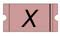Final location: \$start_city Distance traveled: \$distance_string miles (\$segment_text)
_EOS_ push(@html, \$html) ; return join("", @html) ; } sub get_missed_html { my(\$segment_id, \$num_missed, \$skill_id, \$skillset, @part_array) = @_; my(\$html, @html); my(\$problem_html) = &get_missed_answer_html(\$skill_id, @part_array); @html = () ; \$html = createPageHeader("Math Journey", "", "", "", "Math Journey", "", "", "") ; push(@html, \$html) ; \$html =<<" _EOS_" ;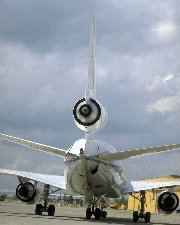\$problem_html
_EOS_ push(@html, \$html) ; return join("", @html) ; } sub get_train_html { my(\$segment_id, \$num_missed, \$skill_id, \$skillset, @part_array) = @_; my(\$html, @html, \$form_html); my(\$code) = &get_skill_code(\$skill_id); my(\$problem_type) = substr(\$code, 0, 3); my(\$problem_level) = substr(\$code, 3, 1); my(\$start_city) = &get_start_city(\$segment_id); my(\$end_city) = &get_end_city(\$segment_id); my(\$xticket_html) = ''; \$xticket_html x= \$num_missed; my(\$remaining_segments) = 30 - \$segment_id; my(\$missed_text); my(\$scene_image) = ""; my(\$distance) = &get_miles(\$segment_id); my(\$distance_remaining) = &get_miles(30) - \$distance; my(\$distance_string) = &commaize(\$distance); my(\$distance_remaining_string) = &commaize(\$distance_remaining); my(\$segment_text) = \$segment_id . " segments"; if (\$segment_id == 1) { \$segment_text = "1 segment"; } my(\$remaining_segment_text) = \$remaining_segments . " segments"; if (\$remaining_segments == 1) { \$remaining_segment_text = "1 segment"; # Bug fixed, ltang, 01/31/2008 } if ( (\$segment_id == 0) || (\$segment_id == 1) || (\$segment_id == 3) || (\$segment_id == 6) || (\$segment_id == 8) || (\$segment_id == 10) || (\$segment_id == 16) || (\$segment_id == 18) || (\$segment_id == 19) || (\$segment_id == 20) || (\$segment_id == 21) || (\$segment_id == 27) || (\$segment_id == 29)) { \$scene_image = ''; } if (\$num_missed == 0) { \$missed_text = "No missed flights"; } elsif (\$num_missed == 1) { \$missed_text = "1 missed flight"; } else { \$missed_text = "" . \$num_missed . " missed flights"; } if (\$problem_type eq "add") { \$form_html = &get_train_html_add(\$segment_id, \$num_missed, \$problem_level, \$skillset, @part_array); } elsif (\$problem_type eq "sub") { \$form_html = &get_train_html_sub(\$segment_id, \$num_missed, \$problem_level, \$skillset, @part_array); } elsif (\$problem_type eq "mul") { \$form_html = &get_train_html_mul(\$segment_id, \$num_missed, \$problem_level, \$skillset, @part_array); } elsif (\$problem_type eq "div") { \$form_html = &get_train_html_div(\$segment_id, \$num_missed, \$problem_level, \$skillset, @part_array); } elsif (\$problem_type eq "rou") { \$form_html = &get_train_html_rou(\$segment_id, \$num_missed, \$problem_level, \$skillset, @part_array); } @html = () ; \$html = createPageHeader("Math Journey", "", "", "", "Math Journey", "", "", "") ; push(@html, \$html) ; \$html =<<" _EOS_" ;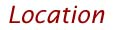\$start_city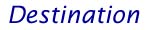\$end_city
\$scene_image
 Distance traveled: \$distance_string miles (\$segment_text) Distance remaining: \$distance_remaining_string miles (\$remaining_segment_text) \$missed_text \$xticket_html Start over

\$form_html
_EOS_ push(@html, \$html) ; return join("", @html) ; } sub get_train_html_add { my(\$segment_id, \$num_missed, \$problem_level, \$skillset, @part_array) = @_; my(\$html); \$html = ' ' . &commaize(\$part_array) . ' + ' . &commaize(\$part_array) . ' = ?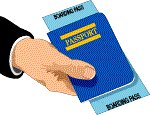To board the flight, enter the correct answer:
'; return \$html; } sub get_train_html_sub { my(\$segment_id, \$num_missed, \$problem_level, \$skillset, @part_array) = @_; my(\$html); \$html = ' ' . &commaize(\$part_array) . ' - ' . &commaize(\$part_array) . ' = ?To board the flight, enter the correct answer:
'; return \$html; } sub get_train_html_mul { my(\$segment_id, \$num_missed, \$problem_level, \$skillset, @part_array) = @_; my(\$html); \$html = ' ' . &commaize(\$part_array) . ' X ' . &commaize(\$part_array) . ' = ?To board the flight, enter the correct answer:
'; return \$html; } sub get_train_html_div { my(\$segment_id, \$num_missed, \$problem_level, \$skillset, @part_array) = @_; my(\$html); \$html = ' ' . &commaize(\$part_array) . ' ÷ ' . &commaize(\$part_array) . ' = ?To board the flight, enter the correct answer: r
'; return \$html; } sub get_train_html_rou { my(\$segment_id, \$num_missed, \$problem_level, \$skillset, @part_array) = @_; my(\$html); \$html = ' What is ' . &commaize(\$part_array) . ' rounded to the nearest ' . \$part_array . '?To board the flight, enter the correct answer:
`; push(@html, \$html) ; if ((! defined(\$help)) || (\$help eq "")) { \$help = "/faq.html"; } if (defined(\$up) && (\$up ne "")) { \$up = '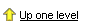'; } # #Keep the following banner text close and tight. Don't add any extra new lines #unless absolutely necesssary. Thanks! #-dk 8-2-00 # \$html =qq` `; push(@html, \$html) ; # # Add Quia IXL ad banner below the Quia banner # -ltang 02/25/08 # \$banner=IXL_AD(); \$html=qq'`; push(@html, \$html) ; \$html=qq`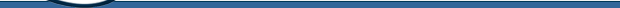\$bar_title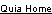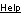\$login  \$auxmenu`; push(@html, \$html); if(defined \$adText) { \$html=qq``; push(@html, \$html); } \$html=qq`